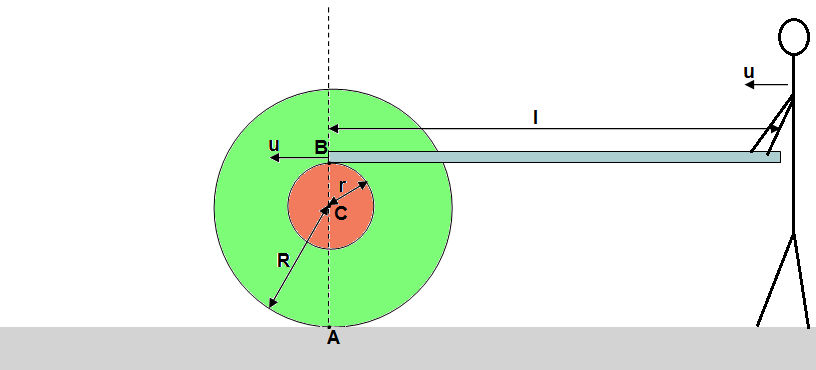#### You may also like### Cannon Balls

How high will a ball taking a million seconds to fall travel?### Dangerous Driver?

Was it possible that this dangerous driving penalty was issued in error?### Speedo

Investigate the relationship between speeds recorded and the distance travelled in this kinematic scenario.

# Board and Spool

##### Age 16 to 18Challenge Level

A man is holding one end of a board and the another end is on a spool with the outer radius $R$ and the inner radius $r$. A board does not slip on the spool and the spool does not slip on the ground. The man starts to move with the board with a speed $u$.1) How long does it take for the man to reach the spool?

2) Find the distance which must be traveled by the man to reach the spool.

3) Find the distance traveled by the man if $r = R$.

4) Calculate the time and the distance if $l = 298\mathrm{cm}$, $R = 101\mathrm{cm}$, $r = 86\mathrm{cm}$, $u = 1\mathrm{m/s}$.